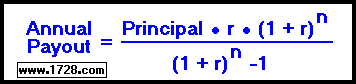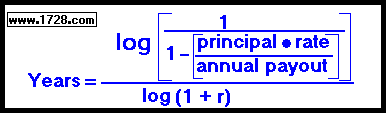Annuity Payout FormulasTo see how an annuity gets paid out, let's use a short period of time.

An annuity has a \$50,000 principal, a 7% rate and a 3 year payout period. How much is each annual payout?

payout = \$50,000 • .07 • (1 + .07)3 ÷ [ (1 + .07)3 -1 ]

payout = 3,500 • 1.225043 ÷ [ 1.225043 -1 ]

payout = 4,287.65 ÷ [ .225043 ]

annual payout = 19,052.58

Now that we have the annual payout amount, let's see how the payout process works.

At the end of the first year, the \$50,000 principal has earned \$3,500.00 interest (50,000 × .07 = \$3,500.00) increasing the annuity balance to \$53,500. After the first annual payout of \$19,052.58, the balance is reduced to \$34,447.42.

At the end of the second year, the \$34,447.42 balance has earned \$2,411.32 interest (\$34,447.42 × .07 = \$2,411.32) increasing the annuity balance to \$36,858.74. After the second annual payout, the balance is reduced to \$17,806.16.

At the end of the third year, the \$17,806.16 balance has earned \$1,246.43 interest (\$17,806.16 × .07 = \$1,246.43) increasing the annuity balance to \$19,052.59. Now when the third annual payout is made, the balance is reduced to zero. (Okay, the balance is .01 but that's close enough).

Looking at the mathematics involved with a manually calculated payout, it's much easier to use the calculator isn't it?Upon retirement, you'd like to have an annuity that will pay out \$25,000.00 per year for 20 years. If the annuity interest rate is 8 per cent, how much principal would you need? Principal = (25,000 • [(1.08)20 -1)] ÷ (.08 • (1.08)20 Principal = (25,000 • 3.6609571438) ÷ (.08 • 4.6609571438) Principal = 91,523.93 ÷ 0.3728765715 Principal = 245,453.69You are retiring with a 7% \$300,000.00 annuity that pays 50,000.00 per year. How long will this annuity last? Years = log (1 ÷ (1 - [300,000 • .07 ÷ 50,000])) ÷ log (1.07) Years = log (1 ÷ (1 - .42)) ÷ 0.0293837777 Years = log (1 ÷ .58) ÷ 0.0293837777 Years = log (1.724137931) ÷ 0.0293837777 Years = 0.2365720064 ÷ 0.0293837777 Years = 8.051109322

RETURN   TO   THE   ANNUITY   PAYOUT CALCULATOR

RETURN   TO   FINANCIAL   CALCULATORS

RETURN   TO   HOME   PAGE